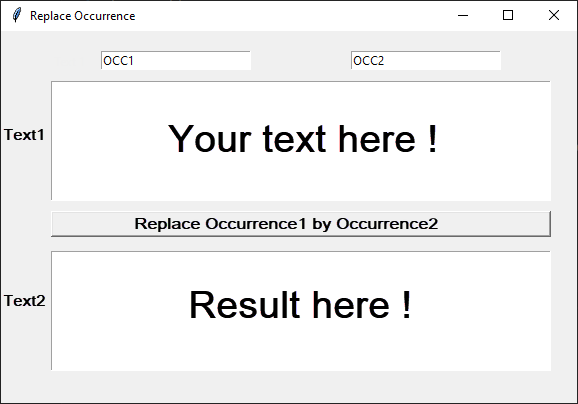# Solution Exercise 204 : Text Replacement GUI with Tkinter in Python

Nov 5, 2023#### Exercise 204

Write a Python program based on the Tkinter library that prompts the user to enter text content in a text area T1 and an occurrence occ1 they want to replace with an occurrence occ2 in the text T1 and display the result in the text area T2, as shown in the figure above.

#### Solution

```from tkinter import *

# Function that performs the replacement action
def replace():

# Get data from the occ1 and occ2 entry fields
occurrence1 = occ1.get()
occurrence2 = occ2.get()

# Get the content of the text area T1
s1 = T1.get("1.0", END)

# Replace occurrence1 with occurrence2
# and save the text in a variable s2
s2 = s1.replace(occurrence1, occurrence2)

# Insert the new text s2 into the text field T2
T2.delete("1.0", END)  # Clear previous content in T2
T2.insert("1.0", s2)

# Create the main application window
root = Tk()
root.title("Replace Occurrence")
root.geometry("800x600")

# Create entry fields for occurrences occ1 and occ2
occ1 = Entry(root)
occ1.insert(0, 'occ1')  # Default text in occ1
occ1.place(x=100, y=20, width=200)
occ2 = Entry(root)
occ2.insert(0, 'occ2')  # Default text in occ2
occ2.place(x=400, y=20, width=200)

# Create the label for text area T1
TL1 = Label(root, text="Text 1")
TL1.place(x=50, y=20)

# Create the text area T1 for input
T1 = Text(root)
T1.place(x=50, y=50, width=700, height=200)

# Create the button that performs the action
btn_replace = Button(root,
text='Replace Occurrence1 by Occurrence2',
command=replace)
btn_replace.place(x=100, y=270, width=650)

# Create the label for text area T2
TL2 = Label(root, text="Text 2")
TL2.place(x=50, y=270)

# Create the text area T2 for output
T2 = Text(root)
T2.place(x=50, y=310, width=700, height=200)

# Start the Tkinter event loop
root.mainloop()```

Younes Derfoufi
my-courses.net